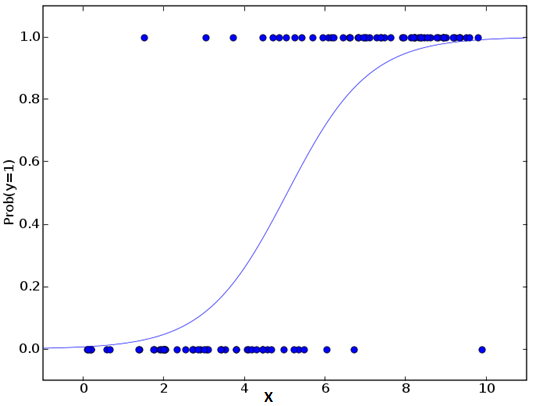# A brief idea on Logistic Regression

Logistic regression is used to find the probability of event=Success and event=Failure. We should use logistic regression when the dependent variable is binary (0/ 1, True/ False, Yes/ No) in nature. Here the value of Y ranges from 0 to 1 and it can represented by following equation.

odds= p/ (1-p) = probability of event occurrence / probability of not event occurrence ln(odds) = ln(p/(1-p)) logit§ = ln(p/(1-p)) = b0+b1X1+b2X2+b3X3…+bkXk

Above, p is the probability of presence of the characteristic of interest. A question that you should ask here is “why have we used log in the equation?”.

Since we are working here with a binomial distribution (dependent variable), we need to choose a link function which is best suited for this distribution. And, it is logit function. In the equation above, the parameters are chosen to maximize the likelihood of observing the sample values rather than minimizing the sum of squared errors (like in ordinary regression).#### Important Points:

• Logistic regression is widely used for classification problems
• Logistic regression doesn’t require linear relationship between dependent and independent variables. It can handle various types of relationships because it applies a non-linear log transformation to the predicted odds ratio
• To avoid over fitting and under fitting, we should include all significant variables. A good approach to ensure this practice is to use a step wise method to estimate the logistic regression
• It requires large sample sizes because maximum likelihood estimates are less powerful at low sample sizes than ordinary least square
• The independent variables should not be correlated with each other i.e. no multi collinearity . However, we have the options to include interaction effects of categorical variables in the analysis and in the model.
• If the values of dependent variable is ordinal, then it is called as Ordinal logistic regression
• If dependent variable is multi class then it is known as Multinomial Logistic regression .